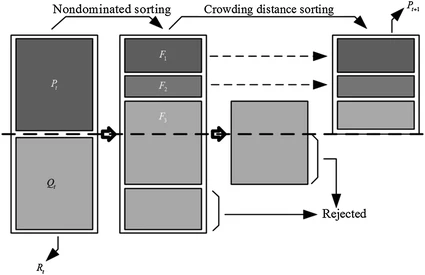Book Chapter

# Chaotic system modelling using a neural network with optimized structure

By
Lamamra K.
Vaidyanathan S.
Azar A.T.
Salah C.B.

In this work, the Artificial Neural Networks (ANN) are used to model a chaotic system. A method based on the Non-dominated Sorting Genetic Algorithm II (NSGA-II) is used to determine the best parameters of a Multilayer Perceptron (MLP) artificial neural network. Using NSGA-II, the optimal connection weights between the input layer and the hidden layer are obtained. Using NSGA-II, the connection weights between the hidden layer and the output layer are also obtained. This ensures the necessary learning to the neural network. The optimized functions by NSGA-II are the number of neurons in the hidden layer of MLP and the modelling error between the desired output and the output of the neural model. After the construction and training of the neural model, the selected model is used for the prediction of the chaotic system behaviour. This method is applied to model the chaotic system of Mackey-Glass time series prediction problem. Simulation results are presented to illustrate the proposed methodology. © Springer International Publishing AG 2017. All rights reserved.